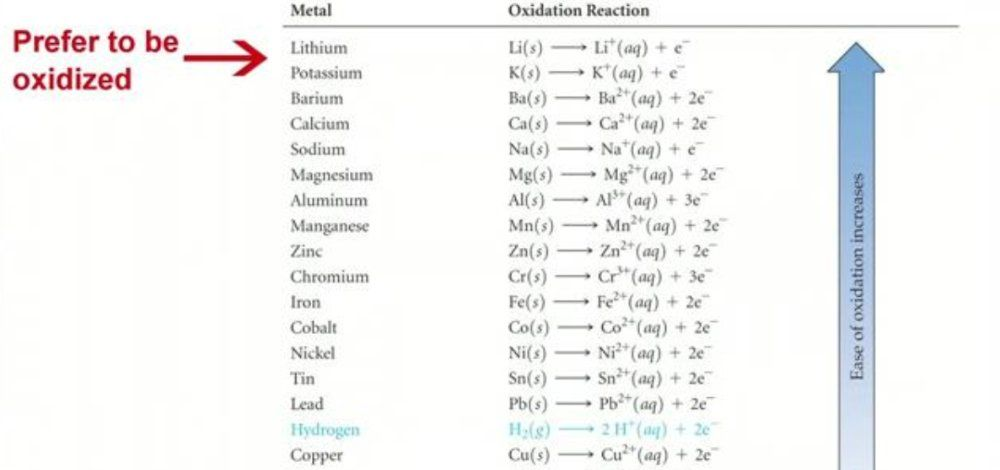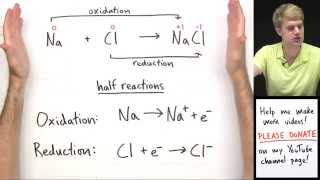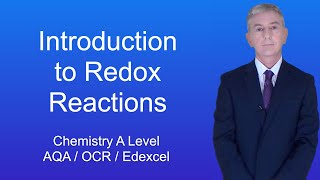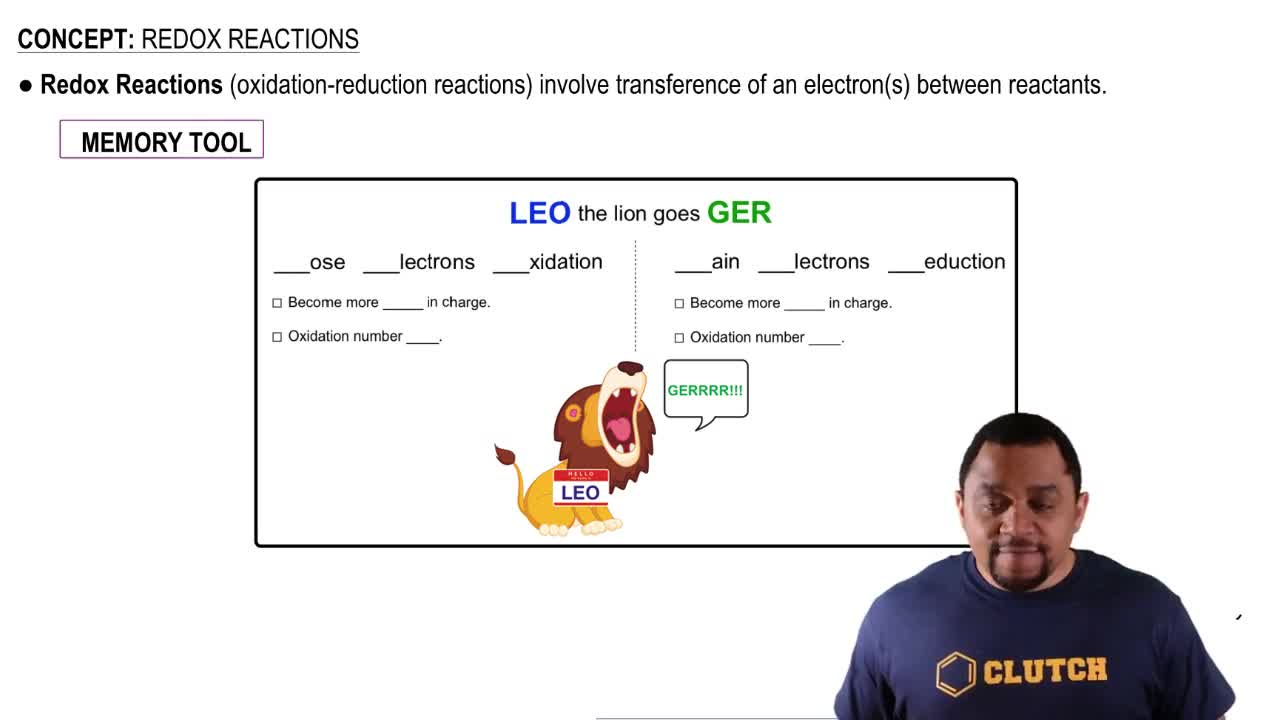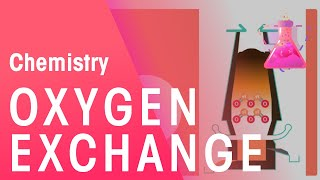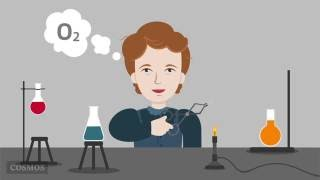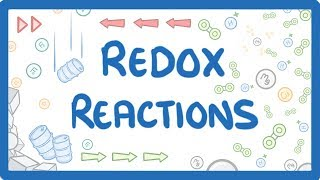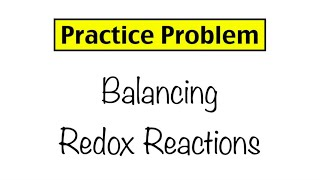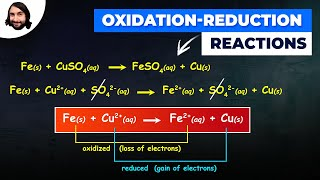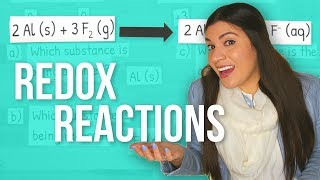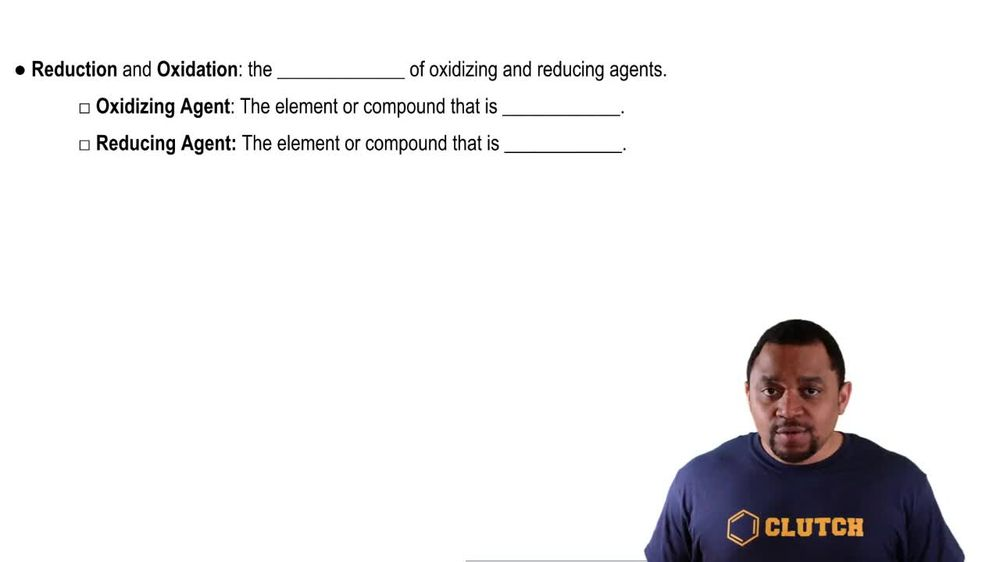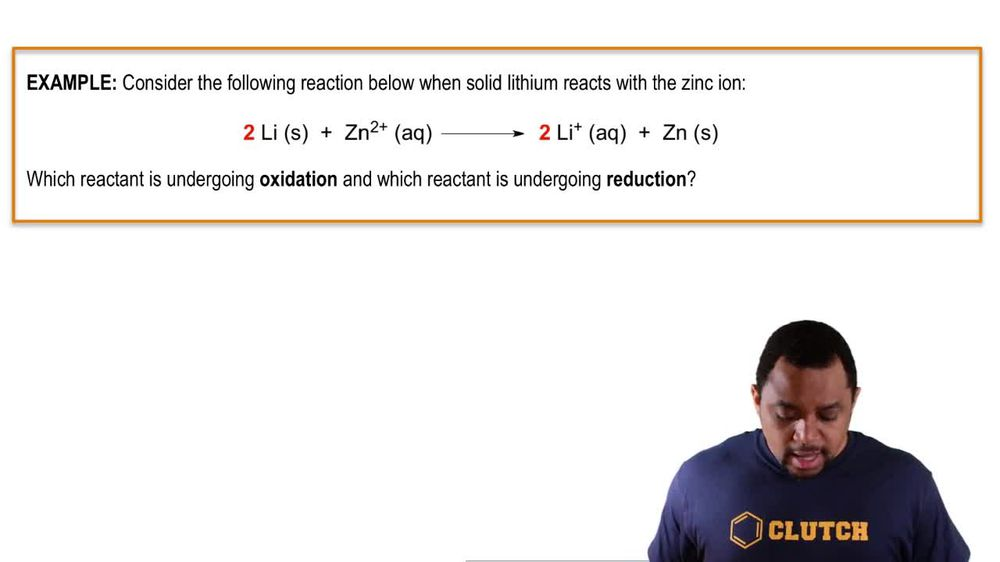Start typing, then use the up and down arrows to select an option from the list.
1. 6. Chemical Quantities & Aqueous Reactions2. Redox Reactions# Redox Reactions Example 1

by Jules Bruno
122 views
1
1
1
in this example question, it says. Consider the following reaction below when solid lithium reacts with the zinc ion. So here we have two moles of lithium solid reacting with one mole of zinc ion in its acquiesce state, and it produces two moles of lithium ion in its acquiesced eight plus zinc solid. The question says, which reactant is undergoing oxidation and which reacting is undergoing reduction. So if we take a look here, lithium solid is in its natural state, So lithium solid oxidation number initially is zero. Zinc here is in its ionic form. It has its charge present. Remember, if we have the charge of the ion, then that can equal the oxidation number. So here this will be plus two going to the other side. We have lithium ion again. It's in its ionic form. If the charge is present, then that charges connected to the oxidation number, so it's plus one and then zinc is now in its natural state, so it's zero. So when we're talking about what's been oxidized, what's been reduced, we look at the reactions. So we have lithium solid and we have what zinc two plus lithium as a reactant goes from having an oxidation number of zero to an oxidation number of plus one. We're gonna say that it's oxidation. Number went up, It increased. It went from zero to plus one. Remember, if you're oxidation number increases, that means that you've been oxidized, So lithium solid has been oxidized. Zinc zinc starts off with plus two as it's oxidation number. And then as a product, it goes down to zero. We see that it's oxidation number decreased. Remember, if you're oxidation number decreased, you've been reduced. So zinc two plus ion has been reduced. Remember what's been oxidized and reduced is the reacted. We look on the product side just to see how our oxidation number has changed. But again, it is the reactant that are oxidized or reduced. In this case, it would give us lithium solid as being oxidized and zinc two plus ion as being reduced